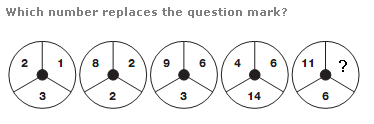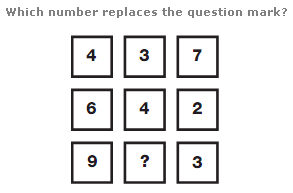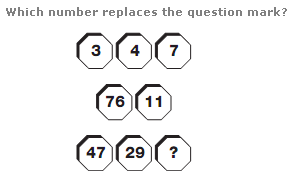# Puzzles - Number puzzles

### Exercise :: Number puzzlesAnswer : 13 Explanation : As you move from left to right, the sum of the digits in each circle forms a sequence of multiples of 6, from 6 to 30.Answer : 6 Explanation : Working in rows, the central value equals the difference between the left and right hand values.Answer : 18 Explanation : Starting on the top left, and moving clockwise around the diagram, add the first two values together to give the next value along. Repeat this sequence.